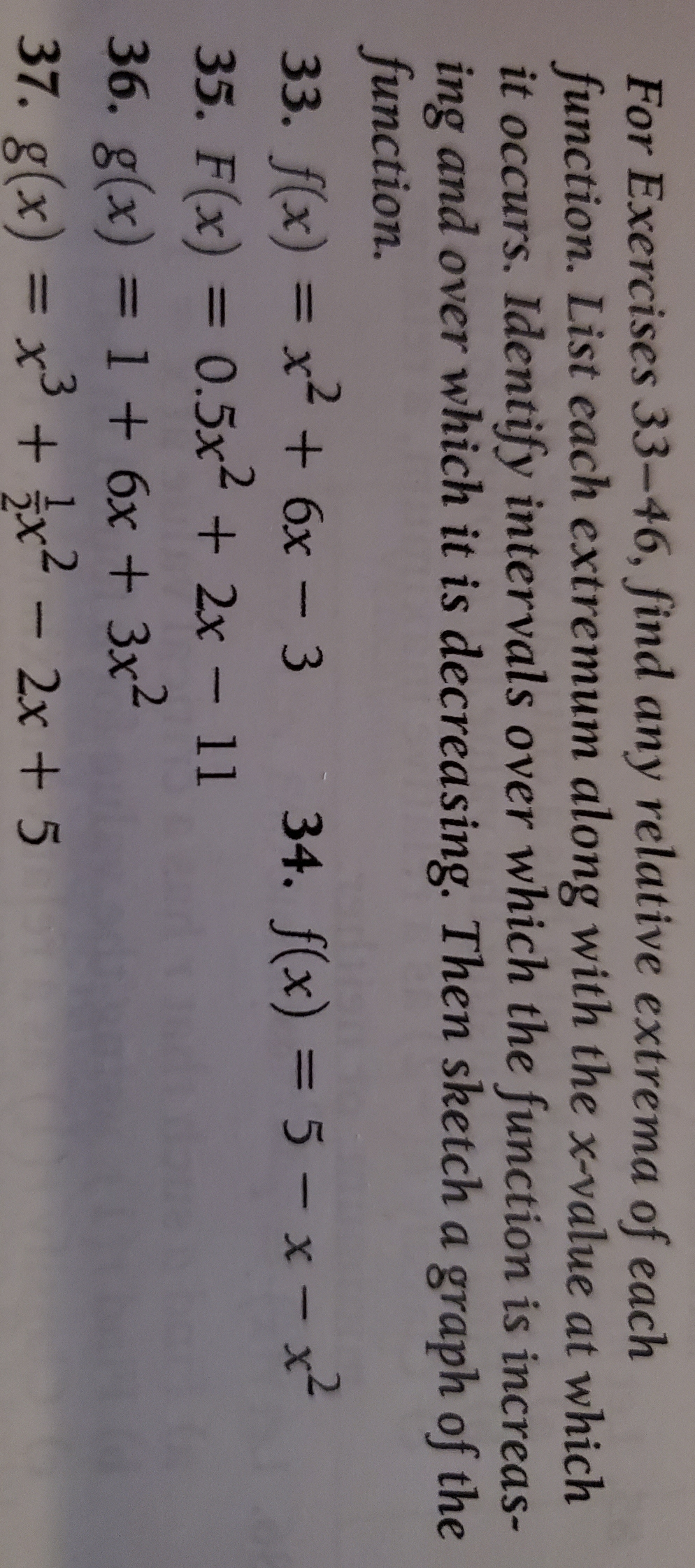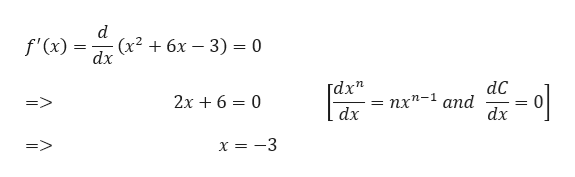# For Exercises 33-46, find any relative extrema of eachfunction. List each extremum along with the x-value at whichit occurs. Identify intervals over which the function is increas -ing and over which it is decreasing. Then sketch a graph of thefunction.33. f(x) = x +6x- 3 34. f(x) = 5 - x- x35. F(x) = 0.5x2 2x- 1136. g(x) = 1 +6x + 3x4-37. g(x) = x3 + }x22x+ 5

Question
69 views

Questions: 33, 35, 37

Thankshelp_outlineImage TranscriptioncloseFor Exercises 33-46, find any relative extrema of each function. List each extremum along with the x-value at which it occurs. Identify intervals over which the function is increas - ing and over which it is decreasing. Then sketch a graph of the function. 33. f(x) = x +6x- 3 34. f(x) = 5 - x- x 35. F(x) = 0.5x2 2x- 11 36. g(x) = 1 +6x + 3x4 - 37. g(x) = x3 + }x2 2x+ 5 fullscreen
check_circle

Step 1

Note: Hello there! You seem to have submitted multiple questions. As per our policy, we will solve only first question for you. If you need help in solving the other questions, then kindly upload them again separately. One of our experts will solve them for you.

Step 2

We need to find the relative maximum and minimum values of f(x), where f(x) is given by,

Step 3

Let’s find out the critical points of f(x). For that we n...help_outlineImage Transcriptionclose(x? + 6х — 3) %3 0 dx f'(x) = dC - пх*-1 and dx 2х + 6 — 0 dx x = -3 fullscreen

### Want to see the full answer?

See Solution

#### Want to see this answer and more?

Solutions are written by subject experts who are available 24/7. Questions are typically answered within 1 hour.*

See Solution
*Response times may vary by subject and question.
Tagged in

### Other